# Polynomial Evaluation VI

## LabVIEW 2018 Help

Edition Date: March 2018
Part Number: 371361R-01
View Product InfoLabVIEW 2016 HelpLabVIEW 2017 HelpLabVIEW 2018 HelpLabVIEW 2019 HelpLabVIEW 2020 Help

Owning Palette: Polynomial VIs

Requires: Full Development System

Evaluates the polynomial P(x) with a single value or multiple values. The data types you wire to the a and P(x) inputs determine the polymorphic instance to use.

Use the pull-down menu to select an instance of this VI.

 Select an instance Scalar Polynomial Evaluation (DBL)Scalar Polynomial Evaluation (CDB)1D Polynomial Evaluation (DBL)1D Polynomial Evaluation (CDB)2D Polynomial Evaluation (DBL)2D Polynomial Evaluation (CDB)

## Scalar Polynomial Evaluation (DBL)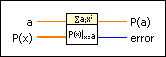a specifies the real value at which the VI evaluates P(x).P(x) contains the real polynomial coefficients in ascending order of power.P(a) returns the result of evaluating P(x) at a.error returns any error or warning from the VI. You can wire error to the Error Cluster From Error Code VI to convert the error code or warning into an error cluster.

## Scalar Polynomial Evaluation (CDB)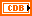a specifies the complex value at which the VI evaluates P(x).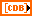P(x) contains the complex polynomial coefficients in ascending order of power.P(a) returns the result of evaluating P(x) at a.error returns any error or warning from the VI. You can wire error to the Error Cluster From Error Code VI to convert the error code or warning into an error cluster.

## 1D Polynomial Evaluation (DBL)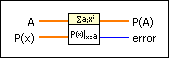A is an array of real values.P(x) contains the real polynomial coefficients in ascending order of power.P(A) returns the result of evaluating P(x) on an element-by-element basis with the values in A.error returns any error or warning from the VI. You can wire error to the Error Cluster From Error Code VI to convert the error code or warning into an error cluster.

## 1D Polynomial Evaluation (CDB)A is an array of complex values.P(x) contains the complex polynomial coefficients in ascending order of power.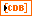P(A) returns the result of evaluating P(x) on an element-by-element basis with the values in A.error returns any error or warning from the VI. You can wire error to the Error Cluster From Error Code VI to convert the error code or warning into an error cluster.

## 2D Polynomial Evaluation (DBL)A is a matrix of real values.P(x) contains the real polynomial coefficients in ascending order of power.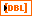P(A) returns the result of evaluating P(x) on an element-by-element basis with the values in A.error returns any error or warning from the VI. You can wire error to the Error Cluster From Error Code VI to convert the error code or warning into an error cluster.

## 2D Polynomial Evaluation (CDB)A is a matrix of complex values.P(x) contains the complex polynomial coefficients in ascending order of power.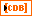P(A) returns the result of evaluating P(x) on an element-by-element basis with the values in A.error returns any error or warning from the VI. You can wire error to the Error Cluster From Error Code VI to convert the error code or warning into an error cluster.